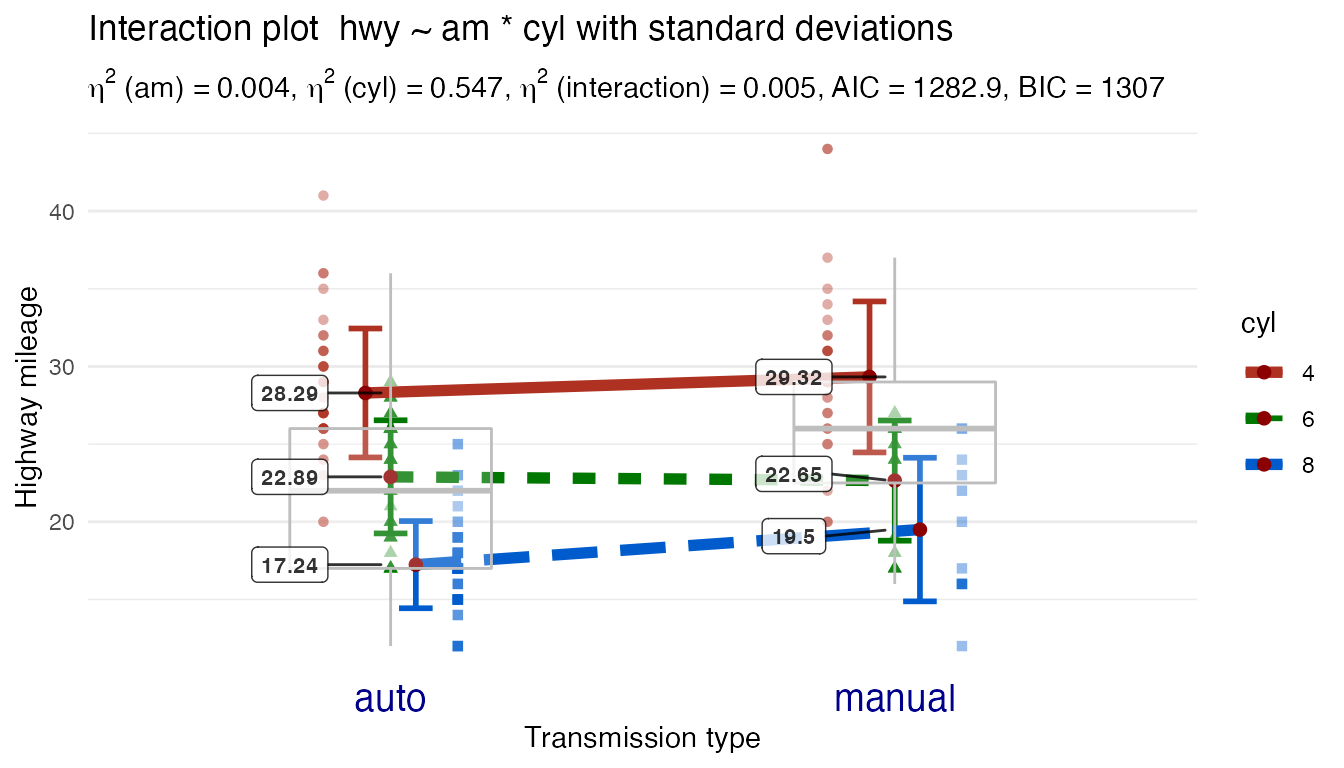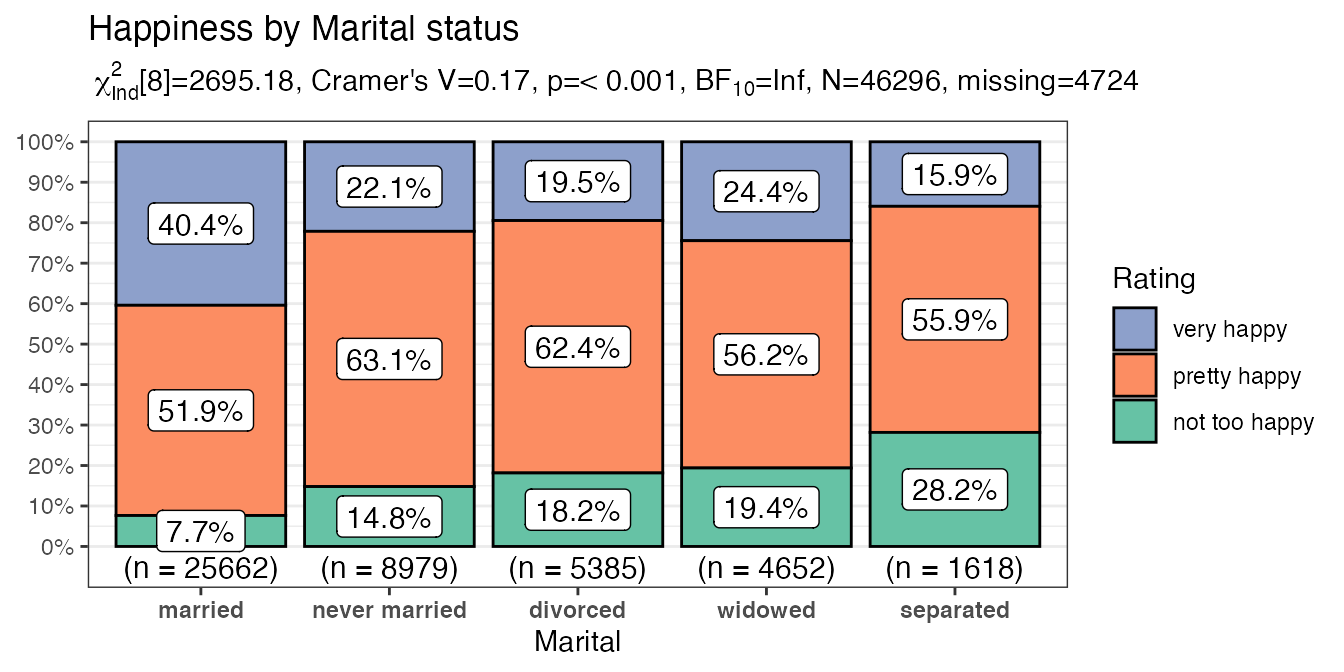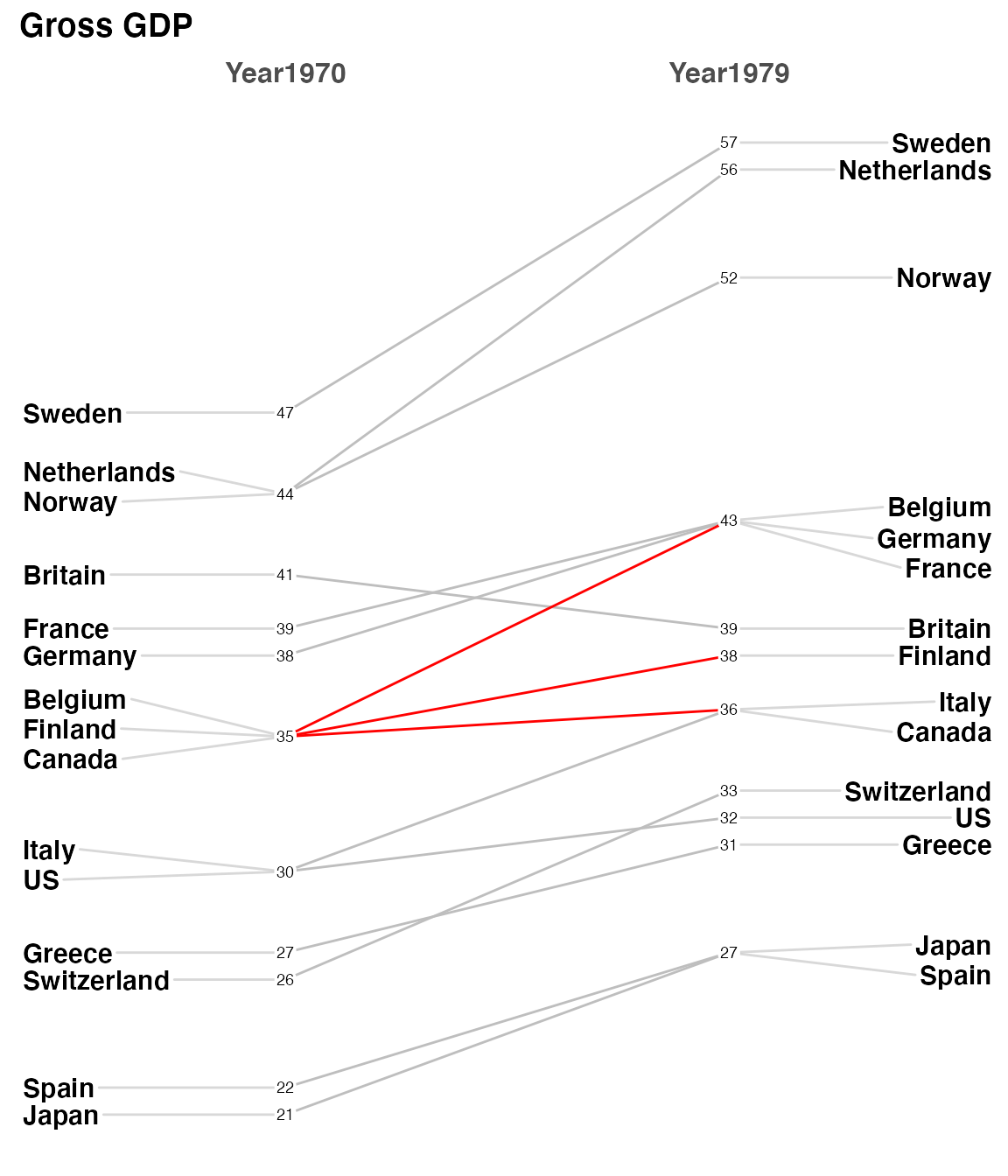### Overview

A package that includes functions that I find useful for teaching statistics as well as actually practicing the art. They typically are not “new” methods but rather wrappers around either base R or other packages and concepts I’m trying to master. Currently contains:

• `Plot2WayANOVA` which as the name implies conducts a 2 way ANOVA and plots the results using `ggplot2`
• `PlotXTabs` which as the name implies plots cross tabulated variables using `ggplot2`
• `PlotXTabs2` which wraps around ggplot2 to provide Bivariate bar charts for categorical and ordinal data.
• `newggslopegraph` which creates a Tufte“esque” slopegraph using `ggplot2`
• `chaid_table` provides tabular summary of `CHAID` `partykit` object
• `cross2_var_vectors` a helper function to build lists of “crossed” variables
• `Mode` which finds the modal value(s) in a vector of data
• `SeeDist` which wraps around ggplot2 to provide visualizations of univariate data.
• `OurConf` is a simulation function that helps you learn about confidence intervals

### Installation

``````# Install from CRAN
install.packages("CGPfunctions")

# Or the development version from GitHub
# install.packages("devtools")
devtools::install_github("ibecav/CGPfunctions")``````

### Usage

#### Example Plot2WayANOVA plotExample Plot2WayANOVA

#### Example PlotXTabs2 plotExample PlotXTabs2 plot

#### Example newggslopegraph plotExample newggslopegraph plot

### Credits

Many thanks to Danielle Navarro and the book Learning Statistics with R.

### Leaving Feedback

If you like CGPfunctions, please consider leaving feedback here.

### Contributing

Contributions in the form of feedback, comments, code, and bug reports are most welcome. How to contribute:

• Issues, bug reports, and wish lists: File a GitHub issue.
• Contact the maintainer ibecav at gmail.com by email.